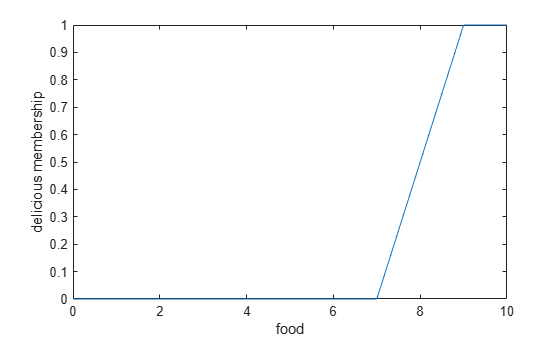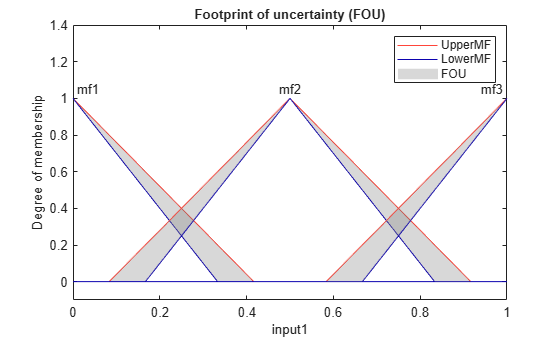Documentation

# plotmf

Plot membership functions for input or output variable

## Syntax

``plotmf(fis,variableType,variableIndex)``
``plotmf(___,numPoints)``
``[xOut,mfOut] = plotmf(___)``
``[xOut,umfOut,lmfOut] = plotmf(___)``

## Description

example

````plotmf(fis,variableType,variableIndex)` plots the membership functions for an input or output variable in the fuzzy inference system `fis`.```

example

````plotmf(___,numPoints)` specifies the number of data points to plot for each membership function.```

example

````[xOut,mfOut] = plotmf(___)` returns the universe of discourse (`xOut`) and membership function (`mfOut`) values without plotting them. Use this syntax when `fis` is a type-1 fuzzy inference system.```

example

````[xOut,umfOut,lmfOut] = plotmf(___)` returns the universe of discourse (`xOut`), upper membership function (`umfOut`), and lower membership function (`lmfOut`) values without plotting them. Use this syntax when `fis` is a type-2 fuzzy inference system.```

## Examples

collapse all

Create a fuzzy inference system.

`fis = readfis('tipper');`

Plot the membership functions for the first input variable.

`plotmf(fis,'input',1)`Create a fuzzy inference system.

`fis = readfis('tipper');`

Plot the membership functions for the first output variable using `101` data points for each membership function.

`plotmf(fis,'output',1,101)`Create a fuzzy inference system.

`fis = readfis('tipper');`

Obtain the x-axis and y-axis data for the membership functions of the second input variable.

`[xOut,yOut] = plotmf(fis,'input',2);`

You can then, for example, plot a single membership function using this data.

```plot(xOut(:,2),yOut(:,2)) xlabel('food') ylabel('delicious membership')```Create a type-2 fuzzy inference system.

`fis = mamfistype2('NumInputs',3,'NumOutputs',1);`

Plot the membership functions for the second input variable.

`plotmf(fis,'input',1)`The type-2 membership functions have a footprint of uncertainty (FOU) between their upper and lower membership functions.

You can also obtain the plotting data without generating a plot.

`[xOut,umfOut,lmfOut] = plotmf(fis,'input',1);`

You can then plot individual membership functions or plot the data using your own custom formatting. For example, plot the upper and lower membership functions for only the second membership function of the first input variable.

```plot(xOut(:,2),umfOut(:,2),'r',xOut(:,2),lmfOut(:,2),'b') xlabel('input1') ylabel('delicious membership')```## Input Arguments

collapse all

Fuzzy inference system, specified as one of the following:

`plotmf` does not support plotting output membership functions of Sugeno systems.

Variable type, specified as one of the following:

• `'input'` — Input variable

• `'output'` — Output variable

Variable index, specified as a positive integer. If `variableType` is:

• `'input'`, then `variableIndex` must be less than or equal to the number of input variables in `fis`

• `'output'`, then `variableIndex` must be less than or equal to the number of output variables in `fis`

Number of data points to plot, specified as a positive integer.

## Output Arguments

collapse all

Universe of discourse data, returned as a `numPoints`-by-NMF array, where NMF is the number of membership functions for the variable specified by `variableType` and `variableIndex`.

Membership function data for a type-1 membership function, returned as a `numPoints`-by-NMF array, where NMF is the number of membership functions for the variable specified by `variableType` and `variableIndex`.

Upper membership function data for a type-2 membership function, returned as a `numPoints`-by-NMF array, where NMF is the number of membership functions for the variable specified by `variableType` and `variableIndex`.

Lower membership function data for a type-2 membership function, returned as a `numPoints`-by-NMF array, where NMF is the number of membership functions for the variable specified by `variableType` and `variableIndex`.

## Compatibility Considerations

expand all

Warns starting in R2019b